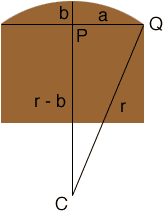Quandaries and Queries Hi. I was constructing my fence and was having some problems trying to do a perfect (or as close as possible) arch.  If i know a section of a fence is for example 85 inches wide and I want a 4 inch rise from the top rail how do i figure out the radius?         --- ----------------- [                   ] [                   ] [                   ]   This would be the fence with the long horizontal line been where the rail is, and the small horizontal line been where i want the top of arch to reach.   Thank You Andres Hi Andres, I drew a diagram of a section of your fence. C is the center of the circle, r is the radius of the circle, a is half the width of the section and b is the height of the rise. In the example you sent a is 85/2 = 42.5 inches and b is 4 inches.On the diagram, triangle QPC is a right triangle and hence, by Pythagoras' Theorem a2 + (r - b)2 = r2 That is a2 + r2 - 2br + b2 = r2  Solving for r gives r = (a2 + b2)/2b With your dimensions I get r = (42.52 + 42)/8 = 227.78 inches Penny Go to Math Central